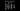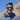Blog# Backpropagation

### August 31, 2018This may be the most important post in the series, and also the most overlooked, both for the same reason - this is where the maths gets interesting! It is important to get to grips with it when looking at deep learning algorithms - although later you may never have to implement it manually,thanks to the power of deep learning frameworks, understanding it is crucial when debugging your model.

Don’t fret though, the principles we applied in the Learning by Gradient Descent post will help us a great deal to really understand this.

But before we dive into the maths, it makes sense to describe what the backpropagation algorithm is.

Backpropagation is an algorithm for computing the partial derivatives of the parameters, by going through the network backwards, layer by layer, starting from the output layer.

## Why backpropagation to learn the weights?

It first makes sense to understand why we use backprop to compute derivatives in the first place. Aren’t there better alternatives?

What about numerically approximating the gradient by seeing how much the cost changes directly when we nudge each parameter? Well, there are often tens of thousands, if not more parameters, and computing cost using forward prop individually for all of them is very expensive.

So we have to analytically compute our partial derivative through the chain rule.

If we were to go through the network forwards, and consider the weights from the first layer, we’d have to consider how a nudge affects the immediate outputs - i.e. the 2nd layer, and then how that nudge in the 2nd layer propagates to the 3rd layer etc. For the 2nd layer we’d have to recompute all the later layer calculations, which is expensive since the later layers’ partial derivatives are used again. And again, for the weights in the 3rd layer, and so on.

To put the maths formally:

$\frac{\partial{J}}{\partial{W^{}}} = \frac{\partial{A^{}}}{\partial{W^{}}}*\frac{\partial{A^{}}}{\partial{A^{}}}*[\frac{\partial{A^{}}}{\partial{A^{}}}...\frac{\partial{A^{[L]}}}{\partial{A^{[L-1]}}}*\frac{\partial{J}}{\partial{A^{[L]}}}]$

$\frac{\partial{J}}{\partial{W^{}}} = \frac{\partial{A^{}}}{\partial{W^{}}}*[\frac{\partial{A^{}}}{\partial{A^{}}}...\frac{\partial{A^{[L]}}}{\partial{A^{[L-1]}}}*\frac{\partial{J}}{\partial{A^{[L]}}}]$

Notice how the term in square brackets (the ripple effect of the “nudges” from the 3rd layer onwards) is the same for both equations. So what if we computed the term first, then computed the weights for the first and second layer?

More generally, when computing the weights in layers $1...l$ we’ll be reusing the same computations involving partial derivatives from layer $l+1$ onwards.

So it makes sense to start from the output, and make one pass backward, computing the partial derivatives layer by layer - each layer’s calculations being reused in subsequent calculations, such that we don’t compute anything twice.

Aside: Linking this to other areas of computer science as a way of looking at the underlying principles, this is an example of the more general principle of bottom-up dynamic programming.

## Deriving the Backpropagation Algorithm

When deriving the algorithm’s equations, we will intersperse the intuition with the maths.

It is helpful to restate the tips given in the Learning by Gradient Descent post:

• Partial Derivative Intuition: Think of $\frac{\partial{y}}{\partial{x}}$ loosely as quantifying how much $y$ would change if you gave the value of $x$ a little “nudge” at that point.

• Breaking down computations - we can use the chain rule to aid us in our computation - rather than trying to compute the derivative in one fell swoop, we break up the computation into smaller intermediate steps.

• Computing the chain rule - when thinking about which intermediate values to include in our chain rule expression, think about the immediate outputs of equations involving $x$ - which other values get directly affected when we slightly nudge $x$?

• One element at a time - rather than worrying about the entire matrix $A$, we’ll instead look at an element $A_{ij}$. One equation we will refer to time and time again is:

$C_{ij} = \sum_k A_{ik}B_{kj} \iff C=A.B$

A useful tip when trying to go from one element to a matrix is to look for summations over repeated indices (here it was k) - this suggests a matrix multiplication.

Another useful equation is the element-wise product of two matrices:

$C_{ij} = A_{ij}B_{ij} \iff C=A*B$

• Sanity check the dimensions - check the dimensions of the matrices all match (the derivative matrix should have same dimensions as the original matrix, and all matrices being multiplied together should have dimensions that align.

### Backpropagating through layer l:

Let’s break the backprop into a smaller computation, that of one particular layer $l$. Let’s assume we have computed $\frac{\partial{J}}{\partial{A^{[l]}}}$ - in the overall algorithm this gets propagated back from layer $l+1$.

We need to compute the relative partial derivatives for this layer so it makes sense to remind ourselves of the equations from the forward pass:

$Z^{[l]} = W^{[l]}.A^{[l-1]} + b^{[l]}$

$A^{[l]} = g(Z^{[l]})$

First let’s consider how to compute $\frac{\partial{J}}{\partial{Z^{[l]}}}$. Consider a single element $Z^{[l]}_{ij}$ - since we are applying the activation function $g(x)$ individually to each element in the matrix, a nudge in $Z^{[l]}_{ij}$ will only affect the corresponding activated output - $A^{[l]}_{ij}$. The magnitude of the nudge of $A_{ij}$ is by definition the derivative of the function $g(x)$ at the value of $Z_{ij}$. So we have:

$\frac{dA^{[l]}_{ij}}{dZ^{[l]}_{ij}} = g'(Z^{[l]}_{ij})$

We can apply the chain rule:

$\frac{\partial{J}}{\partial{Z^{[l]}_{ij}}} = \frac{\partial{J}}{\partial{A^{[l]}_{ij}}} * g'(Z^{[l]}_{ij})$

This is just an element-wise product of two matrices - since we are multiplying the corresponding indices $_{ij}$ of both matrices together. So we have our equation:

$\frac{\partial{J}}{\partial{Z^{[l]}}} = \frac{\partial{J}}{\partial{A^{[l]}}}*g'(Z^{[L]})$

As a sanity check, $\frac{\partial{J}}{\partial{A^{[l]}}}$ has the same dimensions as $A^{[l]}$ which has dimensions $n_l$ x $m$, which is the same as $g'(Z^{[L]})$ since it is applying a function element-wise so preserves dimensions of $Z^{[L]}$. So the dimensions of $\frac{\partial{J}}{\partial{Z^{[l]}}}$ do match with $Z^{[L]}$.

Brilliant! Next, let’s look at the effect of nudging the weight $W^{[l]}_{jk}$. To make it easier to conceptualise, let’s rewrite the matrices in terms at an individual neuron level:

$z^{[l](i)}_j = \sum_{k=1}^{n} W^{[l]}_{jk} a^{[l-1](i)}_k + b^{[l]}_j$

This nudge in $W^{[l]}_{jk}$ affects the weighted input of the neuron $z^{[l]}_{j}$ across all examples in the training set - we will take the average gradient across the examples. The magnitude of the nudge to the value of neuron $z^{[l]}_{j}$ is - $a^{[l-1]}_{k}$ times the nudge of $W^{[l]}_{jk}$ since $W^{[l]}_{jk}$ is multiplied by $a^{[l-1]}_{k}$ in the equation above.

Also consider a nudge in $b^{[l]}_{j}$, the magnitude of the corresponding nudge to $z^{[l]}_{j}$ will be the same, and just like with $W^{[l]}_{jk}$ we will average the effect of the nudge across the examples. So we have:

$\frac{\partial{J}}{\partial{W^{[l]}_{jk}}} = \frac{1}{m} \sum_{i=1}^{m}\frac{\partial{J}}{\partial{z^{[l](i)}_{j}}}*\frac{\partial{z^{[l](i)}_{j}}}{\partial{W^{[l]}_{jk}}} = \frac{1}{m} \sum_{i=1}^{m}\frac{\partial{J}}{\partial{z^{[l](i)}_{j}}} * a^{[l-1](i)}_{k}$

$\frac{\partial{J}}{\partial{b^{[l]}_{j}}} = \frac{1}{m} \sum_{i=1}^{m}\frac{\partial{J}}{\partial{z^{[l](i)}_{j}}}*\frac{\partial{z^{[l](i)}_{j}}}{\partial{b^{[l]}_{j}}} = \frac{1}{m} \sum_{i=1}^{m}\frac{\partial{J}}{\partial{z^{[l](i)}_{j}}}$

It’s worth noting though how similar these equations are to logistic regression - just that instead of $x$ we have the more general $a^{[l-1]}$. As we mentioned in the previous post, the principles for logistic regression are just scaled and generalised for a feedforward neural network.

We can now switch our notation back to considering the matrices, having gained the insight from looking at one neuron.

$\frac{\partial{J}}{\partial{W^{[l]}_{jk}}} = \frac{1}{m} \sum_{i=1}^{m}\frac{\partial{J}}{\partial{Z^{[l]}_{ji}}} * A^{[l-1]}_{ki} = \frac{1}{m} \sum_{i=1}^{m}\frac{\partial{J}}{\partial{Z^{[l]}_{ji}}} * A^{[l-1]T}_{ik}$

This gives us our equations:

$\frac{\partial{J}}{\partial{W^{[l]}_{jk}}}= \frac{1}{m} \frac{\partial{J}}{\partial{Z^{[l]}}}.A^{[l-1]T}$

As a sanity check: the right-hand-side multiplies a $n_l$ x $m$ matrix with a $m$ x $n_{l-1}$ matrix, giving a $n_{l}$ x $n_{l-1}$ matrix - so the dimensions do match.

$\frac{\partial{J}}{\partial{b^{[l]}}} = \frac{1}{m} \sum_{i=1}^{m}\frac{\partial{J}}{\partial{Z^{[l](i)}}}$

Finally, we just need to consider how the gradient propagates to layer $l-1$ - we didn’t have to consider this in the specific case of logistic regression as there was only one layer.

Again, the intuition about partial derivatives as “nudges” will help us. Let’s consider one neuron in layer $l-1$ for the $i^{th}$ example: $a^{[l-1](i)}_k$. A nudge in this neuron affects all of the neurons’ weighted inputs for that example in the next layer, so we will have to sum partial derivatives across the neurons. The magnitude of the nudge in the weighted input $z^{[l](i)}_j$ will be the original nudge multiplied by the corresponding weight $W^{[l]}_{jk}$. Indeed the equation is:

$\frac{\partial{J}}{\partial{a^{[l-1](i)}_{k}}} =\sum_{j=1}^{n_l}\frac{\partial{J}}{\partial{z^{[l](i)}_{j}}}*\frac{\partial{z^{[l](i)}_{j}}}{\partial{a^{[l-q](i)}_{j}}}= \sum_{j=1}^{n_l}\frac{\partial{J}}{\partial{z^{[l](i)}_{j}}}*W^{[l]}_{jk}$

Again, now switching back to the matrix notation, now that we’ve got the intuition:

$\frac{\partial{J}}{\partial{A^{[l-1]}_{ki}}} = \sum_{j=1}^{n_l}W^{[l]}_{jk}*\frac{\partial{J}}{\partial{z^{[l]}_{ji}}} = \sum_{j=1}^{n_l}W^{[l]T}_{kj}*\frac{\partial{J}}{\partial{z^{[l]}_{ji}}}$

So as a matrix multiplication:

$\frac{\partial{J}}{\partial{A^{[l-1]}}} = W^{[l]T}.\frac{\partial{J}}{\partial{z^{[l]}}}$

Again as a sanity check: the right-hand-side multiplies a $n_{l-1}$ x $n_l$ matrix with a $n_l$ x $m$ matrix, giving a $n_{l-1}$ x $m$ matrix - so the dimensions do match.

Now having looked at the general layer case, let’s look at the final layer of the network. Some potential final layer activations are:

• no activation - for a regression task
• sigmoid - for binary classification
• softmax - for multi-class classification

For regression and binary classification, as we showed before - $\frac{\partial{J}}{\partial{Z^{[L]}}} = \hat{Y} - Y$

It turns out that with softmax for multi-class classification that the same equation holds. As mentioned in the previous post, we will look at the softmax derivation later in the series, when we look at multi-class classification in a convolutional neural network.

In general though for any output layer activation function, you can obtain $\frac{\partial{J}}{\partial{A^{[L]}}}$ from the loss function equation directly, since $A^{[L]} = \hat{Y}$, and then just like in the general case you can compute $g'(Z^{[L]})$ for whichever activation function is used in the output layer and go from there.

And there you have it! You’ve successfully derived backpropagation for a neural network - no mean feat!

To recap, the key equations are:

$\frac{\partial{J}}{\partial{Z^{[l]}}} = \frac{\partial{J}}{\partial{A^{[l]}}}*g'(Z^{[L]})$

$\frac{\partial{J}}{\partial{W^{[l]}}}= \frac{1}{m} \frac{\partial{J}}{\partial{Z^{[l]}}}.A^{[l-1]T}$

$\frac{\partial{J}}{\partial{b^{[l]}}} = \frac{1}{m} \sum_{i=1}^{m}\frac{\partial{J}}{\partial{Z^{[l](i)}}}$

$\frac{\partial{J}}{\partial{A^{[l-1]}}} = W^{[l]T}.\frac{\partial{J}}{\partial{Z^{[l]}}}$

## Code:

We store the intermediate results of the forward pass in a cache, then we store the gradients in another dictionary. The code for the backprop algorithm is just an implementation of the equation we have derived.

Note the activation function used for the intermediate layer is ReLU. The derivative of $ReLU(x)$ is 1 if $x>0$ since it is linear in that region, and 0 otherwise, since the graph is flat there, as we clamp all negative values to zero. NB: technically at $x=0$ the derivative is not defined, but in practice we take it to be 0.

As before, the accompanying code is in the notebook.

.css-1baulvz{display:inline-block;}def backpropagation(cache,Y,parameters):    L = len(parameters)//2    m = Y.shape    grads = {}    grads["dZ" + str(L)]= cache["A" + str(L)] - Y    grads["dW" + str(L)]= (1/m)*np.dot(grads["dZ" + str(L)],cache["A" + str(L-1)].T)    grads["db" + str(L)]= (1/m)*np.sum(grads["dZ" + str(L)],axis=1,keepdims=True)    for l in range(L-1,0,-1):        grads["dA" + str(l)]= np.dot(parameters["W" + str(l+1)].T,grads["dZ" + str(l+1)])        grads["dZ" + str(l)]= np.multiply(grads["dA" + str(l)], relu(cache["Z" + str(l)], deriv = True))        grads["dW" + str(l)]= (1/m)*np.dot(grads["dZ" + str(l)],cache["A" + str(l-1)].T)        grads["db" + str(l)]= (1/m)*np.sum(grads["dZ" + str(l)],axis=1,keepdims=True)    return grads

### Join me on this learning journey!

This summer I’m using my blog to teach the topics I’ve learnt this year. It’s a win-win - you get computer science tutorials and I get to share it with you!

## Conclusion

Let’s take a step backward here and just appreciate the beauty of backpropagation.

What appeared to us as initially a complicated and scary expression for the partial derivatives has been broken down to a layer by layer calculation. With backpropagation we didn’t have to worry about the entire network, we could just look at the local behaviour of a neuron or weight and how the immediate outputs were affected. We could consider all these moving pieces separately, layer by layer, then stitch together the gradients using the chain rule.

When we look at different neural network architectures with specialised layers that differ in layout and functionality, the same principles of backpropagation will still hold - break it down layer by layer, gain intuition about the effect of nudging that parameter and the behaviour within that layer, then finally use chain rule to get the overall expression.